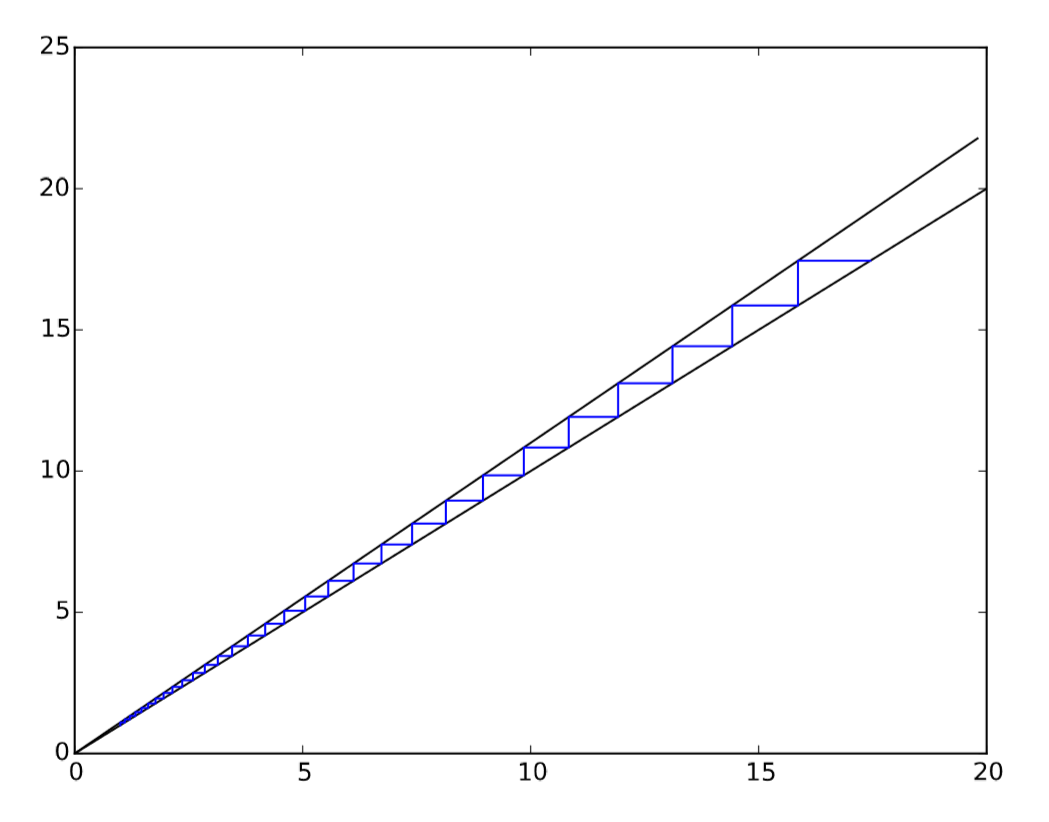$$\newcommand{\id}{\mathrm{id}}$$ $$\newcommand{\Span}{\mathrm{span}}$$ $$\newcommand{\kernel}{\mathrm{null}\,}$$ $$\newcommand{\range}{\mathrm{range}\,}$$ $$\newcommand{\RealPart}{\mathrm{Re}}$$ $$\newcommand{\ImaginaryPart}{\mathrm{Im}}$$ $$\newcommand{\Argument}{\mathrm{Arg}}$$ $$\newcommand{\norm}{\| #1 \|}$$ $$\newcommand{\inner}{\langle #1, #2 \rangle}$$ $$\newcommand{\Span}{\mathrm{span}}$$

# 5.4: 5.4 Graph-Based Phase Space Visualization of Discrete-State Discrete-Time Model

$$\newcommand{\vecs}{\overset { \rightharpoonup} {\mathbf{#1}} }$$

$$\newcommand{\vecd}{\overset{-\!-\!\rightharpoonup}{\vphantom{a}\smash {#1}}}$$

The cobweb plot approach discussed above works only for one-dimensional systems, because we can’t embed such plots for any higher dimensional systems in a 3-D physical space. However, this dimensional restriction vanishes if the system’s states are discrete and ﬁnite. For such a system, you can always enumerate all possible state transitions and create the entire phase space of the system as a state-transition graph, which can be visualized reasonably well even within a 2-D visualization space.

Here is an example. Let’s consider the following second-order (i.e., two-dimensional) difference equation:

$x_{t} =x_{t-1}x_{t-2} \mod{6} \label{(5.18)}$

The “mod 6” at the end of the equation means that its right hand side is always a remainder of the division of $$x_{t−1}x_{t−2}$$ by 6. This means that the possible state of $$x$$ is limited only to 0, 1, 2, 3, 4, or 5, i.e., the state of the system $$(x,y)$$ (where $$y$$ is the previousFigure $$\PageIndex{1}$$: Visual output of Code 5.4. This is a cobweb plot of the exponential growth model simulated in Code 4.9.

value of $$x$$) is conﬁned within a ﬁnite set of $$6×6 = 36$$ possible combinations ranging from (0, 0) to (5, 5). It is very easy to enumerate all the 36 states and numerically study which state each of them will transition to. The result of this analysis looks like:

• $$(0,0) → (0,0)$$

• $$(1,0) → (0,1)$$

• $$(2,0) → (0,2)$$

• ...

• $$(3,2) → (0,3)$$

• $$(4,2) → (2,4)$$

• $$(5,2) → (4,5)$$

• ...

• $$(3,5) → (3,3)$$

• $$(4,5) → (2,4)$$

• $$(5,5) → (1,5)$$

By enumerating all the state transitions, we obtain a list of connections between the discrete states, which forms a network, or a graph in mathematical terminology. We will learn more about modeling and analysis of networks later in this textbook, but I can give you a little bit of a preview here. We can use a Python module called NetworkX  to construct and visualize the network1. See Code 5.5.In this example, a network object, named g, is constructed using NetworkX’s DiGraph (directed graph) object class, because the state transitions have a direction from one state to another. I also did some additional tricks to improve the result of the visualization. I split the network into multiple separate connected components using NetworkX’s connected_ components function, and then arranged them in a grid of plots using pylab’s subplot feature. The result is shown in Fig. 5.5.1.

Each of the six networks represent one connected component, which corresponds to one basin of attraction. The directions of transitions are indicated by thick line segments instead of conventional arrowheads, i.e., each transition goes from the thin end to the thick end of a line (although some thick line segments are hidden beneath the nodes in the crowded areas). You can follow the directions of those links to ﬁnd out where the system is going in each basin of attraction. The attractor may be a single state or a dynamic loop. Don’t worry if you don’t understand the details of this sample code. We will discuss how to use NetworkX in more detail later.

Exercise $$\PageIndex{1}$$

Draw a phase space of the following difference equation within the range $$0 ≤ x < 100$$ by modifying Code 5.5:

$x_{t} = x^{x_{t-1}}_{t-1} \ mod{100}\ \label{(5.19)}$